# Algebra II : Real Numbers

## Example Questions

← Previous 1 3

### Example Question #1 : Real Numbers

Using the properties of negative numbers, determine which answer is equivalent to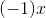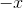Explanation:

Recall the following property of negative numbers: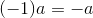It follows that: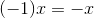### Example Question #2 : Real Numbers

Using the properties of negative numbers, determine which answer is equivalent to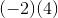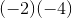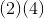Explanation:

Recall the following property of negative numbers: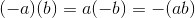It follows that: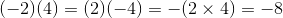### Example Question #3 : Real Numbers

Using the properties of negative numbers, determine which answer is equivalent to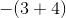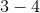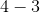All of the other answersExplanation:

Recall the following property of negative numbers: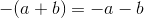It follows that: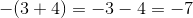### Example Question #1 : Number Theory

Using the properties of negative numbers, determine which answer is equivalent to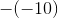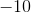Explanation:

Recall the following property of negative numbers: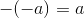It follows that: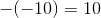### Example Question #1 : Real Numbers

Identify the property of real numbers represented by the following: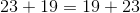Associative Property of Multiplication

Distributive Property

Commutative Property of Multiplication

Explanation:

Recall that the Commutative Propery of Addition states: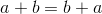It follows that:To help you tell the difference between commutative, associative, and distributive just remember what the words themselves mean.

When you commute you go from one place to another. With commutative properties, numbers are moving from one position to another.

When you associate with someone you are connected or grouped with them. The associative properties involve moving parentheses so that different numbers are grouped together, but they are not changing positions.

When you distribute things you are handing them out. With the distributive property, the number outside the parentheses is being handed out to the numbers inside the parentheses.

### Example Question #2 : Number Theory

Identify the property of real numbers represented by the following: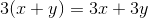Distributive Property

Commutative Property of Multiplication

Associative Property of Multiplication

Distributive Property

Explanation:

Recall that the Distributive Property states: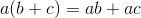It follows that:To help you tell the difference between commutative, associative, and distributive just remember what the words themselves mean.

When you commute you go from one place to another. With commutative properties, numbers are moving from one position to another.

When you associate with someone you are connected or grouped with them. The associative properties involve moving parentheses so that different numbers are grouped together, but they are not changing positions.

When you distribute things you are handing them out. With the distributive property, the number outside the parentheses is being handed out to the numbers inside the parentheses.

### Example Question #5051 : Algebra Ii

Identify the property of real numbers represented by the following: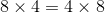Associative Property of Multiplication

Commutative Property of Multiplication

Distributive Property

Commutative Property of Multiplication

Explanation:

Recall that the Commutative Property of Multiplication states: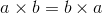It follows that :To help you tell the difference between commutative, associative, and distributive just remember what the words themselves mean.

When you commute you go from one place to another. With commutative properties, numbers are moving from one position to another.

When you associate with someone you are connected or grouped with them. The associative properties involve moving parentheses so that different numbers are grouped together, but they are not changing positions.

When you distribute things you are handing them out. With the distributive property, the number outside the parentheses is being handed out to the numbers inside the parentheses.

### Example Question #1 : Number Theory

Identify the property of real numbers represented by the following: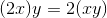Commutative Property of Multiplication

Distributive Property

Associative Property of Multiplication

Associative Property of Multiplication

Explanation:

Recall that the Associative Property of Multiplication states: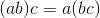It follows that:To help you tell the difference between commutative, associative, and distributive just remember what the words themselves mean.

When you commute you go from one place to another. With commutative properties, numbers are moving from one position to another.

When you associate with someone you are connected or grouped with them. The associative properties involve moving parentheses so that different numbers are grouped together, but they are not changing positions.

When you distribute things you are handing them out. With the distributive property, the number outside the parentheses is being handed out to the numbers inside the parentheses.

### Example Question #5051 : Algebra Ii

Identify the property of real numbers represented by the following: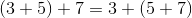Distributive Property

Commutative Property of Multiplication

Associative Property of Multiplication

Explanation:

Recall that the Associative Property of Addition states: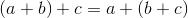It follows that:To help you tell the difference between commutative, associative, and distributive just remember what the words themselves mean.

When you commute you go from one place to another. With commutative properties, numbers are moving from one position to another.

When you associate with someone you are connected or grouped with them. The associative properties involve moving parentheses so that different numbers are grouped together, but they are not changing positions.

When you distribute things you are handing them out. With the distributive property, the number outside the parentheses is being handed out to the numbers inside the parentheses.

### Example Question #3 : Number Theory

Identify the property of real numbers represented by the following: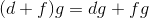Commutative Property of Multiplication

Distributive Property

Associative Property of Multiplication

Distributive Property

Explanation:

Recall that the Distributive Property states:The number outside the parentheses can be on the left or right side of the parentheses, so the following is also true: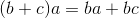It follows that:To help you tell the difference between commutative, associative, and distributive just remember what the words themselves mean.

When you commute you go from one place to another. With commutative properties, numbers are moving from one position to another.

When you associate with someone you are connected or grouped with them. The associative properties involve moving parentheses so that different numbers are grouped together, but they are not changing positions.

When you distribute things you are handing them out. With the distributive property, the number outside the parentheses is being handed out to the numbers inside the parentheses.

← Previous 1 3

### All Algebra II Resources